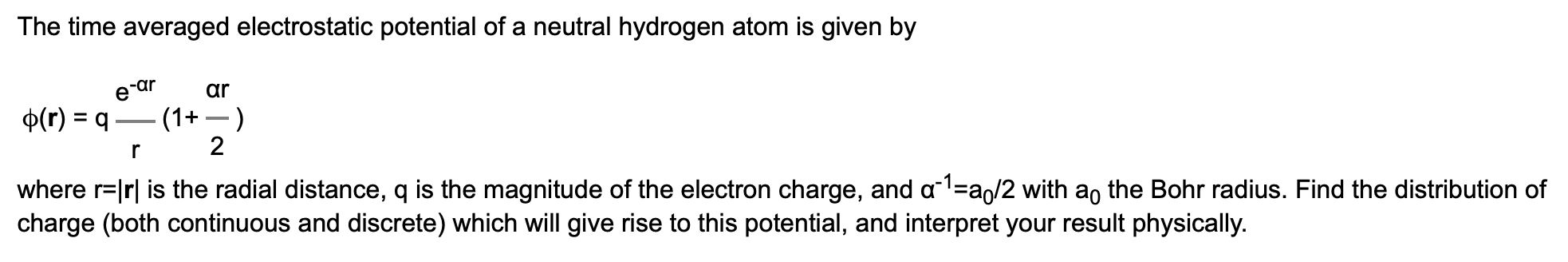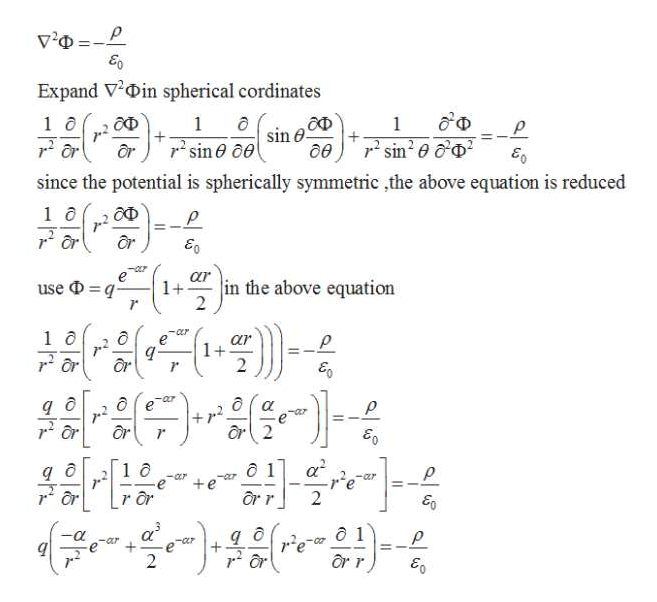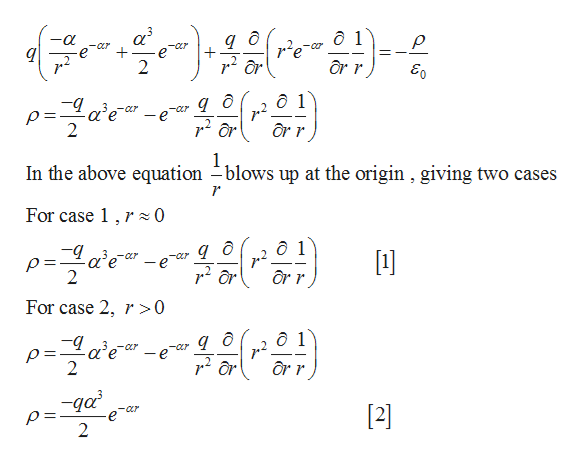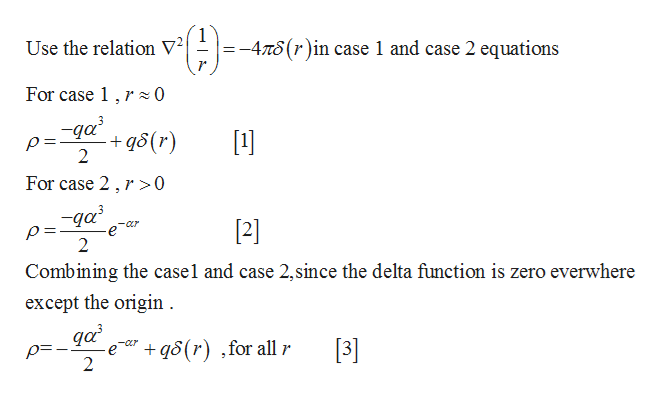# The time averaged electrostatic potential of a neutral hydrogen atom is given byare arp(r) q- (1)2where r lrl is the radial distance, q is the magnitude of the electron charge, and a1 a/2 with ao the Bohr radius. Find the distribution ofcharge (both continuous and discrete) which will give rise to this potential, and interpret your result physically.

Question
3 views

(shown in image)help_outlineImage TranscriptioncloseThe time averaged electrostatic potential of a neutral hydrogen atom is given by ar e ar p(r) q - (1) 2 where r lrl is the radial distance, q is the magnitude of the electron charge, and a1 a/2 with ao the Bohr radius. Find the distribution of charge (both continuous and discrete) which will give rise to this potential, and interpret your result physically. fullscreen
check_circle

Step 1

Write the Poisson equation relating charge density and scalar electric potential.help_outlineImage TranscriptioncloseE0 Expand VDin spherical cordinates 1 sine- 1 6 ОФ 1 sin 0p or since the potential is spherically symmetric ,the above equation is reduced ОФ 2 in the above equation 1+ - use Dq 2 1 o Or 1+ 2 -b а д r2 or e or 2 ô 1 +ea Or r r or 2 a 1 2 ar r fullscreen
Step 2

Continuing  previous step.help_outlineImage Transcriptioncloseд д + -а ô 1 E0 -Cr - ear or r In the above equation -blows up at the origin , giving two cases For case 1 r0 o=2ele" ô 1 er For case 2, r>0 ô 1 r or r -aar -qa CLr p=  e 2 fullscreen
Step 3

Continuing the pre...help_outlineImage TranscriptioncloseUse the relation V2 -4n6(r)in case 1 and case 2 equations For case 1 r z0 -qa +qS(r 2  For case 2,r>0 -qa  Cr е 2 Combining the casel and case 2,since the delta function is zero everwhere except the origin -e q5(r) ,for all r 2  fullscreen

### Want to see the full answer?

See Solution

#### Want to see this answer and more?

Solutions are written by subject experts who are available 24/7. Questions are typically answered within 1 hour.*

See Solution
*Response times may vary by subject and question.
Tagged in

### Other